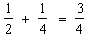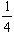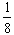Please Support the Monkey! Tell All your Friends and Teachers
 PRACTICAL TEST 2 Section II : Quantitative Section ANSWER EXPLANATIONS We can observe that. We need anotherto bring the total 1. But instead of, the next termissued which is only half of it. This type of error / shortfall occurs, no matter how many terms are added. As the number of terms increase, the sum which comes closer and closer to 1, always remains less than one. Hence B. X < 0 means x is negative. hence x3 is also negative whereas x2 is positive. A positive number is always more than a negative number. Hence B. ( x + y ) is any number between -1 and +1. Hence ( x + y ) can always be made of components x and y, such that x > y or x = y or x < y. Hence D. y2 = 49 implies that y can be either +7 or even -7. Hence y can be equal to 7 or y can be less than 7. ( Since -7 < +7 ) Hence D. Again y2 = 49 implies that y = +7 or -7. If y = +7 then 2y2 < 3y3 If y = -7 then 2y2 being positive will be more than 3y3 which will be negative. Hence D. If a > 0 and b < 0 then a - b will be effectively be the positive sum of the magnitudes of ' a ' and ' b ', whereas a + b will effectively be either a positive or negative difference of the magnitudes of ' a ' and ' b '. Hence A. Test 2 Section 1 : Verbal Section Section 2 : Quantitative Section Section 3 : Analytical Section Section 4 : Quantitative Section Section 5 : Verbal Section Section 6 : Analytical Section Answer Key To Test 2 Answer Explanation To Test 2 Section 1 : Verbal Section Section 2 : Quantitative Section Section 3 : Analytical Section Section 4 : Quantitative Section Section 5 : Verbal Section Section 6 : Analytical Section Test 3